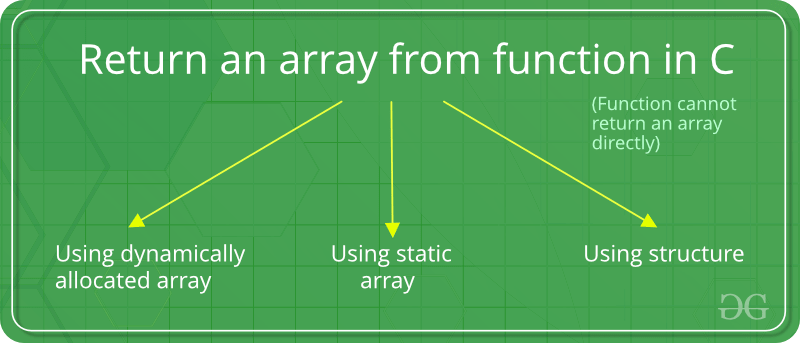# How to return a local array from a C/C++ function?

Consider the below C++ program. Is it right way of returning array from a function?

## C

 `#include ` ` `  `int``* fun() ` `{ ` `    ``int` `arr; ` ` `  `    ``/* Some operations on arr[] */` `    ``arr = 10; ` `    ``arr = 20; ` ` `  `    ``return` `arr; ` `} ` ` `  `int` `main() ` `{ ` `    ``int``* ptr = fun(); ` `    ``printf``(``"%d %d"``, ptr, ptr); ` `    ``return` `0; ` `} `

## C++

 `#include ` `using` `namespace` `std; ` ` `  `int``* fun() ` `{ ` `    ``int` `arr; ` ` `  `    ``/* Some operations on arr[] */` `    ``arr = 10; ` `    ``arr = 20; ` ` `  `    ``return` `arr; ` `} ` ` `  `int` `main() ` `{ ` `    ``int``* ptr = fun(); ` `    ``cout << ptr << ``" "` `<< ptr; ` `    ``return` `0; ` `} `

Warning:

```In function 'int* fun()':
int arr;
^
```
Output:

```10 20
```

The above program is WRONG. It may produce values 10 20 as output or may produce garbage values or may crash. The problem is, we return address of a local variable which is not advised as local variables may not exist in memory after function call is over.

So in simple words, Functions can’t return arrays in C. However, inorder to return the array in C by a function, one of the below alternatives can be used.

Following are some correct ways of returning array:Using Dynamically Allocated Array :

Dynamically allocated memory (allocated using new or malloc()) remains their until we delete it using delete or free(). So we can create a dynamically allocated array and we can delete it once we come out of function.

## C

 `#include ` ` `  `int``* fun(``int` `*arr) ` `{ ` `    ``/* Some operations on arr[] */` `    ``arr = 10; ` `    ``arr = 20; ` ` `  `    ``return` `arr; ` `} ` ` `  `int` `main() ` `{ ` `    ``int` `arr; ` `    ``int``* ptr = fun(arr); ` `    ``printf``(``"%d %d"``, ptr, ptr); ` `    ``return` `0; ` `} `

## C++

 `#include ` `using` `namespace` `std; ` ` `  `int``* fun() ` `{ ` `    ``int``* arr = ``new` `int``; ` ` `  `    ``/* Some operations on arr[] */` `    ``arr = 10; ` `    ``arr = 20; ` ` `  `    ``return` `arr; ` `} ` ` `  `int` `main() ` `{ ` `    ``int``* ptr = fun(); ` `    ``cout << ptr << ``" "` `<< ptr; ` `    ``return` `0; ` `} `

Output:

```10 20
```

Using static array:

Lifetime of a static variable is throughout the program. So we can always create a local static array and return it.

## C

 `#include ` ` `  `int``* fun() ` `{ ` `    ``static` `int` `arr; ` ` `  `    ``/* Some operations on arr[] */` `    ``arr = 10; ` `    ``arr = 20; ` ` `  `    ``return` `arr; ` `} ` ` `  `int` `main() ` `{ ` `    ``int``* ptr = fun(); ` `    ``printf``(``"%d %d"``, ptr, ptr); ` `    ``return` `0; ` `} `

## C++

 `#include ` `using` `namespace` `std; ` ` `  `int``* fun() ` `{ ` `    ``static` `int` `arr; ` ` `  `    ``/* Some operations on arr[] */` `    ``arr = 10; ` `    ``arr = 20; ` ` `  `    ``return` `arr; ` `} ` ` `  `int` `main() ` `{ ` `    ``int``* ptr = fun(); ` `    ``cout << ptr << ``" "` `<< ptr; ` `    ``return` `0; ` `} `

Output:

```10 20
```

Using struct:

We can wrap array in a structure/class and return an instance of the struct/class. The reason for this work is, array members of structures are deeply copied. In below program deep copy happens when we returned instance is copied in main.

## C

 `#include ` ` `  `struct` `arrWrap { ` `    ``int` `arr; ` `}; ` ` `  `struct` `arrWrap fun() ` `{ ` `    ``struct` `arrWrap x; ` ` `  `    ``x.arr = 10; ` `    ``x.arr = 20; ` ` `  `    ``return` `x; ` `} ` ` `  `int` `main() ` `{ ` `    ``struct` `arrWrap x = fun(); ` `    ``printf``(``"%d %d"``, x.arr, x.arr); ` `    ``return` `0; ` `} `

## C++

 `#include ` `using` `namespace` `std; ` ` `  `struct` `arrWrap { ` `    ``int` `arr; ` `}; ` ` `  `struct` `arrWrap fun() ` `{ ` `    ``struct` `arrWrap x; ` ` `  `    ``x.arr = 10; ` `    ``x.arr = 20; ` ` `  `    ``return` `x; ` `} ` ` `  `int` `main() ` `{ ` `    ``struct` `arrWrap x = fun(); ` `    ``cout << x.arr << ``" "` `<< x.arr; ` `    ``return` `0; ` `} `

Output:

```10 20
```

This article is contributed by MAZHAR IMAM KHAN. If you like GeeksforGeeks and would like to contribute, you can also write an article using contribute.geeksforgeeks.org or mail your article to contribute@geeksforgeeks.org. See your article appearing on the GeeksforGeeks main page and help other Geeks.

Don’t stop now and take your learning to the next level. Learn all the important concepts of Data Structures and Algorithms with the help of the most trusted course: DSA Self Paced. Become industry ready at a student-friendly price.

My Personal Notes arrow_drop_up

Improved By : RishabhPrabhu

Article Tags :
Practice Tags :

19

Please write to us at contribute@geeksforgeeks.org to report any issue with the above content.